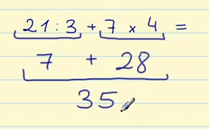Smartick is a fun way to learn math!Nov21

# Combined Operations: How to Solve These Types of Problems

## Combined operations

In this post, we are going to learn the order of operations that you have to follow to do combined operations: addition, subtraction, multiplication, and division. Combined operations cannot be done in a random manner. You have to follow an order:

• Step 1: Do the operations that are inside of the parentheses.

For example:

3 x ( 2 + 4 )

First, we do the operation inside the parentheses: 2 + 4 = 6.

Next, we finish the operation: 3 x 6 = 18.

• Step 2: Do the multiplication and division operations, always working from left to right.

For example:

24 ÷ 6 x 2

First we do the division because it is further to the left than the multiplication: 24 ÷ 6 = 4.

Then we carry out the multiplication operation: 4 x 2 = 8.

• Step 3: Last we do the addition and subtraction.

For example:

2 + 3 x 5

First we do the multiplication: 3 x 5 = 15.

Then we do the addition: 2 + 15 = 17.

We are going to look at an example of combined operations:

6 + ( 8 – 3) x 2

1. First, we do the operation in the parentheses: 8 – 3 = 5. This leaves us with: 6 + 5 x 2.
2. Now we do the multiplication: 5 x 2 = 10.
3. And lastly we are left with the addition operation: 6 + 10 = 16.

Let’s look at another example of combined operations:

21÷ 3 + 7 x 4

1. The first thing to do is do any operations in parenthesis, but in this case, there are not any.
2. The next step is to do the multiplication and division:  21 ÷ 3 = 7 and, on the other side, 7 x 4 = 28.
3. Now we are left with only the addition operation: 7 + 28 = 35.If you want to learn more about combined operations, you can review the previous posts we have written on this subject:

And if you want to learn much more elementary math, join Smartick and keep learning.